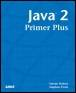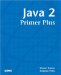LiteralsJava™ 2 Primer PlusBy Steven Haines, Steve Potts Table of Contents Chapter 2.  Keywords, Data Types, and Variables

 A literal represents an actual character, string, or number and not a variable referencing, a character, string, or number in memory. The following are examples of literals: ` 1.0 'c' "Happy" 7 ` In an expression (detailed in the next chapter) you might add 5 to an integer variable: ` int n = a + 5; ` In this case a is a variable and 5 is a literal value. When defining numeric literals there are circumstances where you will have to tell the Java compiler how to interpret the value (what data type it is). When defining a floating-point number such as 1.0, the compiler automatically interprets the value as a double if you want it to be interpreted as a float, you would have to append an f to it. For example: ` float f = 1.0f ` A failure to do so would result in a compilation error stating that you might lose precision in the assignment (because a double is represented by eight bytes and a float is only represented by four).

 TopJava 2 Primer Plus
ISBN: 0672324156
EAN: 2147483647
Year: 2001
Pages: 332

Similar book on Amazon